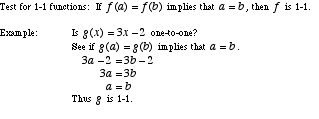index: click on a letter A B C D E F G H I J K L M N O P Q R S T U V W X Y Z A to Z index index: subject areas numbers & symbols sets, logic, proofs geometry algebra trigonometry advanced algebra & pre-calculus calculus advanced topics probability & statistics real world applications multimedia entrieswww.mathwords.com about mathwords website feedback

 One-to-One Function A function for which every element of the range of the function corresponds to exactly one element of the domain. One-to-one is often written 1-1. Note: y = f(x) is a function if it passes the vertical line test. It is a 1-1 function if it passes both the vertical line test and the horizontal line test. Another way of testing whether a function is 1-1 is given below.